Next: Worked example 10.2: Rod Up: Statics Previous: Jointed rods

## Worked example 10.1: Equilibrium of two rods

Question: Suppose that two uniform rods (of negligible thickness) are welded together at right-angles, as shown in the diagram below. Let the first rod be of mass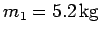and length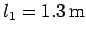. Let the second rod be of mass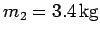and length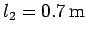. Suppose that the system is suspended from a pivot point located at the free end of the first rod, and then allowed to reach a stable equilibrium state. What angledoes the first rod subtend with the downward vertical in this state?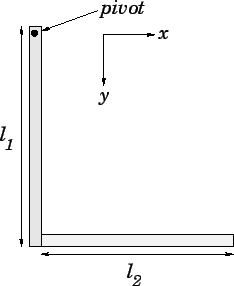Answer: Let us adopt a coordinate system in which the-axis runs parallel to the second rod, whereas the-axis runs parallel to the first. Let the origin of our coordinate system correspond to the pivot point. The centre of mass of the first rod is situated at its mid-point, whose coordinates are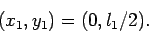Likewise, the centre of mass of the second rod is situated at its mid-point, whose coordinates are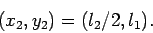It follows that the coordinates of the centre of mass of the whole system are given by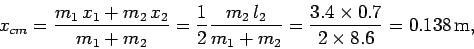and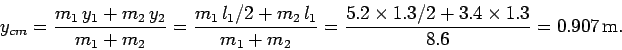The anglesubtended between the line joining the pivot point and the overall centre of mass, and the first rod is simply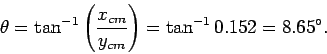When the system reaches a stable equilibrium state then its centre of mass is aligned directly below the pivot point. This implies that the first rod subtends an anglewith the downward vertical.Next: Worked example 10.2: Rod Up: Statics Previous: Jointed rods
Richard Fitzpatrick 2006-02-02